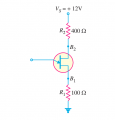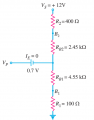# UJT TRANSISTOR

#### aragon1971

Joined Apr 7, 2008
83
hi
i want a help to solve this problemthe n=0.65 and the equivalnce resistance RBB=7kΩ the question is to find the Vp (the volage to turn on the ujt) fisrt
i find the RB1=n RBB and RB2=RBB-RB1 and the equivalance circuit isthe Vp= Vs*(RB1+R1)(/(RBB+R1+R2) ??

Thank you

George

#### ericgibbs

Joined Jan 29, 2010
11,156
hi aragon,
Is this a College assignment.?
E

#### Dodgydave

Joined Jun 22, 2012
9,686
The voltage at the junction of Rb1 and Rb2 is 7.44V , so add 0.7V to get 8.14V total .

#### aragon1971

Joined Apr 7, 2008
83
sorry i must add and the 0.7 Vp=0.7v+ Vs*(RB1+R1)(/(RBB+R1+R2) ??

#### ericgibbs

Joined Jan 29, 2010
11,156
hi aragon,
Second request.

Is this a College assignment.?
E

#### aragon1971

Joined Apr 7, 2008
83
hi aragon,
Is this a College assignment.?
E
yes it is an exercice from a course related to power electronics

#### aragon1971

Joined Apr 7, 2008
83
The voltage at the junction of Rb1 and Rb2 is 7.44V , so add 0.7V to get 8.14V total .
Vp=n *VB1B2+0.7V and VB1B2=VS/(R1+R2+RBB)

#### ericgibbs

Joined Jan 29, 2010
11,156
Hi a.
"According to the data sheet the definition (from data sheet) Vp=n * VB1B2+0.7V "

E

#### ericgibbs

Joined Jan 29, 2010
11,156
hi a,
This is an LTSpice simulation of your original transistor UJT.
I would say for demonstration the results are close enough.

E
BTW: You should allow for the lower voltage on B2, due to the 400R, when calculating 'n'

Last edited: